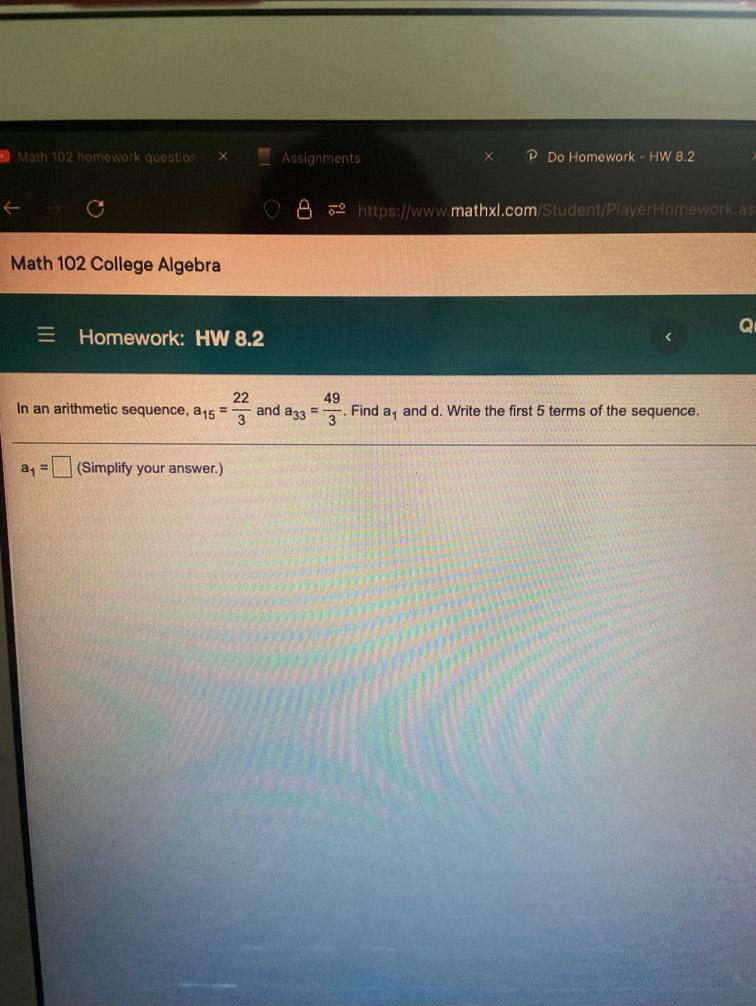Question:

# Math 102 homework quotion Assignments Do Homework - HW 8.2 6- https://www.mathxl.com Student/Player Homework as Math 102 CollegeMath 102 homework quotion Assignments Do Homework - HW 8.2 6- https://www.mathxl.com Student/Player Homework as Math 102 College Algebra QL = Homework: HW 8.2 In an arithmetic sequence, 15 22 49 and a 33 = 3 Find a, and d. Write the first 5 terms of the sequence. an (Simplify your answer.)